09-04-06
How?
Complex Numbers..but there's a bit more to it than that..

..What we do is we..draw a circle around the centre of the complex plane..and count just how long it takes for our equation to throw a complex number outside of that circle..

Huh? ..Don't give up, follow me..

[1..] Basically..

First of all I prepare a piece of paper by drawing lots and lots of regular squares on it.. in a grid pattern..like 'graph paper'..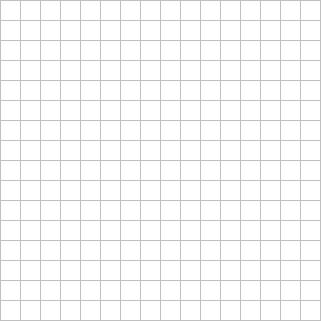[..grid pattern..]

Each square is given it's own unique pair of numbers according to it's position on the grid..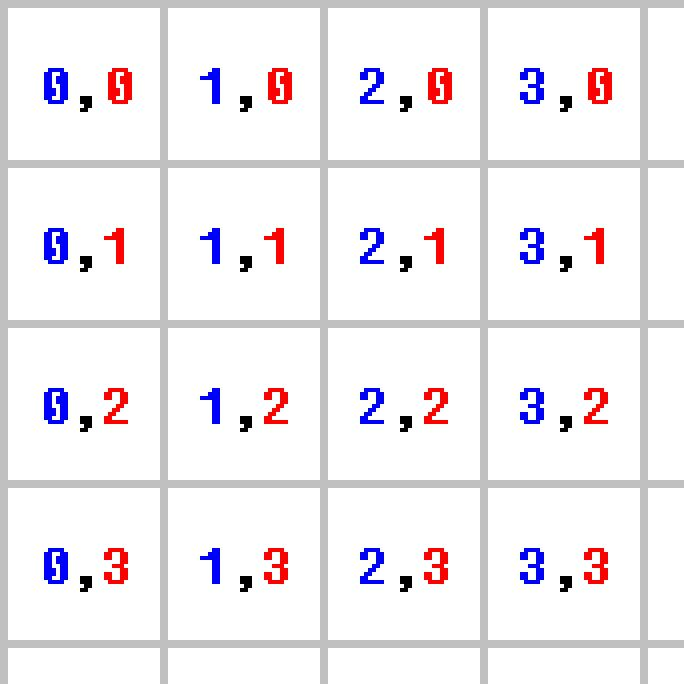[..2-D coordinates..]

I take a pair of numbers from one square, 'feed them into an equation', and the equation comes up with one number..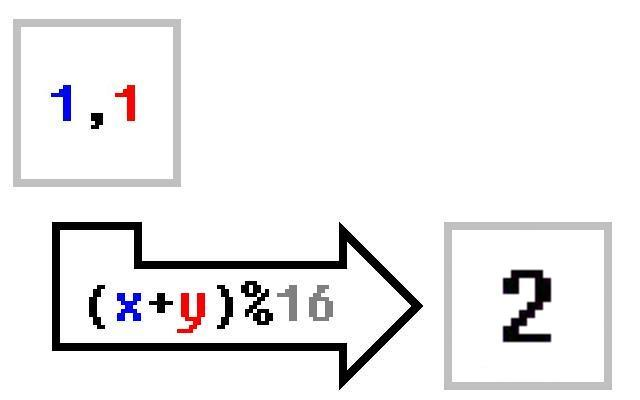[..a simple equation .. (x+y)%16 ..]

If that number is '0' then I colour the square in BLACK; If that number is '1' then I colour the square in MAROON; If the number is '2' then I colour the square in GREEN; etc..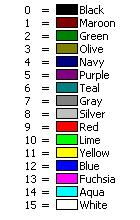[..the palette..]

Then I do the same thing for another square, ..and then another square, ..and then another ..and another ..and another ..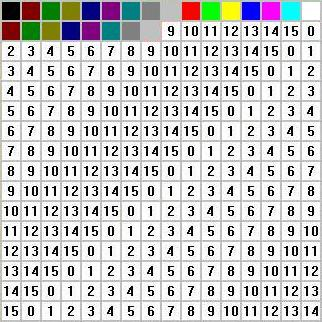[..grid pattern coloured in..]

Well, actually, the grids that I use are usually much too large to be painted by-hand so I write little computer programs to do it all for me..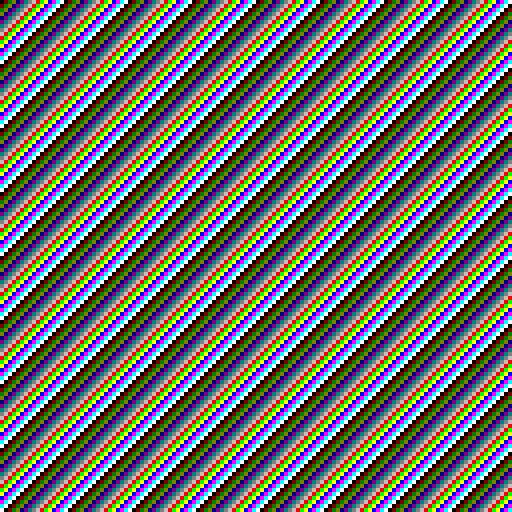[..bigger grid..]

[P.S.] colour = (x + y) % 16 ..

(x + y)  is simple enough, but what's that..that funny '%' symbol, ..and that 16..all about?

Well..the '%' symbol in the equation above means 'modulus'..the 'modulus' is the same as the remainder after division..so, for example,

..4 DIVIDED_BY 4  EQUALS 1 REMAINDER 0;
..5 DIVIDED_BY 4  EQUALS 1 REMAINDER 1;
..6 DIVIDED_BY 4  EQUALS 1 REMAINDER 2;
..7 DIVIDED_BY 4  EQUALS 1 REMAINDER 3;
..8 DIVIDED_BY 4  EQUALS 2 REMAINDER 0;

..4 MODULUS 4 EQUALS 0;
..5 MODULUS 4 EQUALS 1;
..6 MODULUS 4 EQUALS 2;
..7 MODULUS 4 EQUALS 3;
..8 MODULUS 4 EQUALS 0;

Do you see how the remainder, (the 'modulus'), will never be equal to or larger than the number 4? It's the same if we divided by 5..the REMAINDER would never be equal to or larger than the number 5. ..16 was the number we used in the equation above..our palette only has 16 colours. The remainder would never be equal to or larger than 16..anywhere between 0 & 15 in fact, which is just what we want.. Without it, (x+y) could equal ..22, or 55, or 100, or 17, or..or anything..

[2..] 2-D (two-dimensional) Coordinates..

Have you ever used a graphics program like Microsoft Paint before?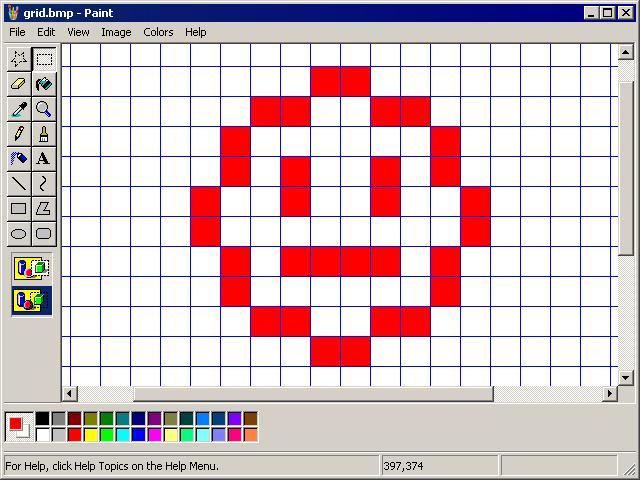[..MS Paint..]

Have you ever noticed the pair of numbers at the bottom of the program, and how those numbers change when you move the mouse?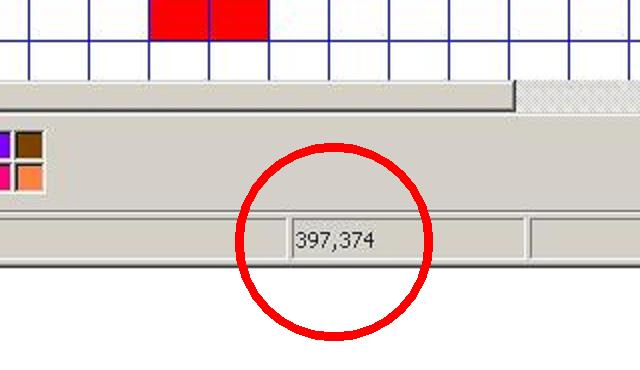[..MS Paint Coordinates..]

When you move the mouse left & right the number on the left-hand-side changes; when you move the mouse up & down the number on the right-hand-side changes.. Those are 'coordinates', (..'2-D coordinates' to be precise..), and they're exactly what I 'feed into' my equations..

..The number on the left-hand-side is usually called 'the X coordinate' and the number on the right-hand-side is usually called 'the Y coordinate'..

[3..] Complex Numbers..

You know how a 2-D coordinate is made up of two numbers (two parts)?

COORDINATE (left_and_right_number , up_and_down_number)

Well there's a strange branch of mathematics called 'complex numbers'. A 'complex number' is made up of two parts too.. In mathematics, one part is called the 'real part', and the other part is called the 'imaginary part'..

COMPLEX_NUMBER (real_part , imaginary_part)

(..I just put the left_and_right_number into the real_part of a complex number and the up_and_down_number into the imaginary_part..and then 'repeatedly run an equation' on it..)
...

Complex numbers can be added together..
COMPLEX_NUMBER (1 , 2) + COMPLEX_NUMBER (3 , 4)
COMPLEX_NUMBER (1 + 3 , 2 + 4)

COMPLEX_NUMBER 4    ,    6   )
Complex numbers can also be multiplied together..
COMPLEX_NUMBER (1 , 2) x COMPLEX_NUMBER (3 , 4)
COMPLEX_NUMBER ( x 3 - 2 x 4 1 x 4 + 2 x 3 )

COMPLEX_NUMBER    3    -     8    ,        +    6    )

COMPLEX_NUMBER (         -         ,           10        )
Strange rule for multiplication?  But that's what makes the pictures so..so, well, complex..

...

..Normal numbers can be described with a number line..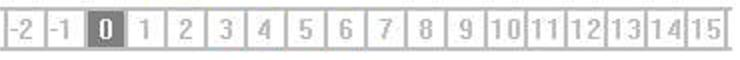[..the number line..]

..Normal addition is like a movement to the right along the number line..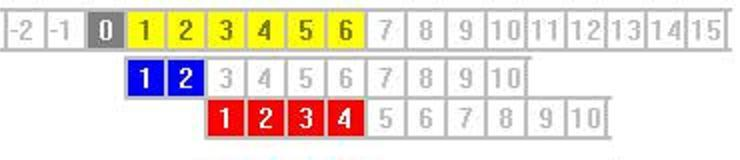2 + 4

..Normal multiplication is like repeated addition..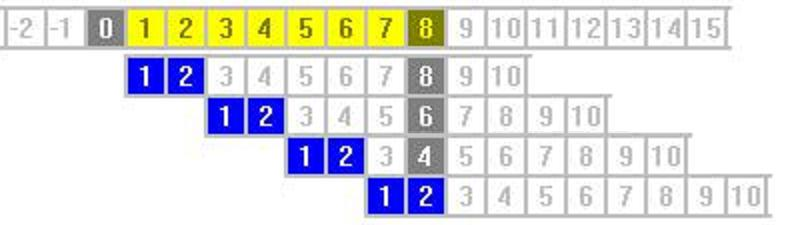2 x 4
.. [normal multipication] ..

You need two number lines to describe complex numbers in this way..one for the real_part, and one for the imaginary_part ..Drawn together, one across the other, mathematician call it the 'complex plane'..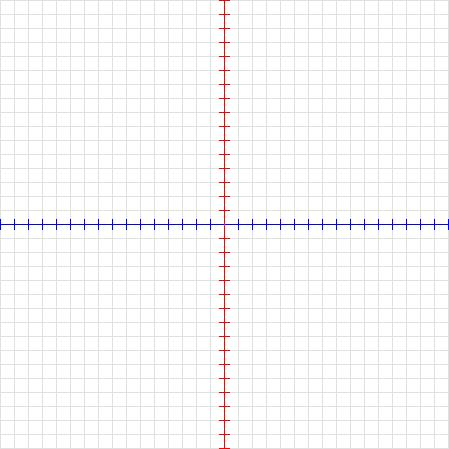[..the complex plane..]

a diagonal movement across the 'complex plane'..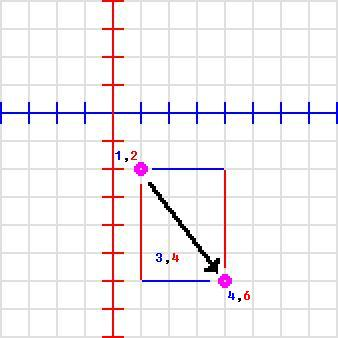COMPLEX_NUMBER (1 , 2) + COMPLEX_NUMBER (3 , 4) = COMPLEX_NUMBER (4 , 6)

..Complex multiplication produces
a 'rotation' and a 'scaling' around the centre of the 'complex plane'..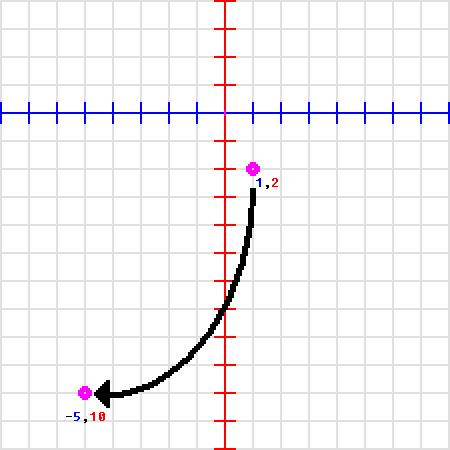COMPLEX_NUMBER (1 , 2) x COMPLEX_NUMBER (3 , 4) = COMPLEX_NUMBER (-5 , 10)

[..complex multiplication..]

Hmm.. Bit lost by that last one? ..OK.. Let's look more closely at exactly what happens when we multiply complex numbers..

..Let's take a complex number, like say ..COMPLEX_NUMBER (1 , 1).. and let's multiply it by another complex number, say, by COMPLEX_NUMBER (1 , 1) again.. What do we get?

COMPLEX_NUMBER (1 , 1) x COMPLEX_NUMBER (1 , 1

COMPLEX_NUMBER (1 x 1 - 1 x 1 , 1 x 1 + 1 x 1

COMPLEX_NUMBER (   1    -    1    ,     1   +    1

COMPLEX_NUMBER (        0          ,         2        )

OK.. ..Let's multiply our answer, COMPLEX_NUMBER (0 , 2), by (1 , 1) yet again.. ..Now what do we get?
COMPLEX_NUMBER (0 , 2) x COMPLEX_NUMBER (1 , 1

COMPLEX_NUMBER (0 x 1 - 2 x 1 , 0 x 1 + 2 x 1

COMPLEX_NUMBER (   0    -    2    ,     0   +   2

COMPLEX_NUMBER (       -2          ,         2          )

Hmm? Let's do it again..and again, and again..(..we'll need a little computer program for this..)

..Starting at..
complex_number = COMPLEX_NUMBER (1 , 1
REPEAT...8 TIMES
complex_number = (   complex_number  x COMPLEX_NUMBER (1 , 1)   )
..What do we get?
 ..   complex_number  COMPLEX_NUMBER    (1 , 1)
 ..    complex_number  COMPLEX_NUMBER   (0 , 2)
 ..    complex_number =  COMPLEX_NUMBER (-2 , 2 )
 ..    complex_number =  COMPLEX_NUMBER (-4 , 0)
 ..    complex_number =  COMPLEX_NUMBER (-4, -4)
 ..    complex_number =  COMPLEX_NUMBER (0 , -8)
 ..    complex_number =  COMPLEX_NUMBER (8 , -8)
 ..    complex_number =  COMPLEX_NUMBER (16 , 0)
 ..    complex_number =  COMPLEX_NUMBER (16 , 16)
..Lets plot the results on a graph..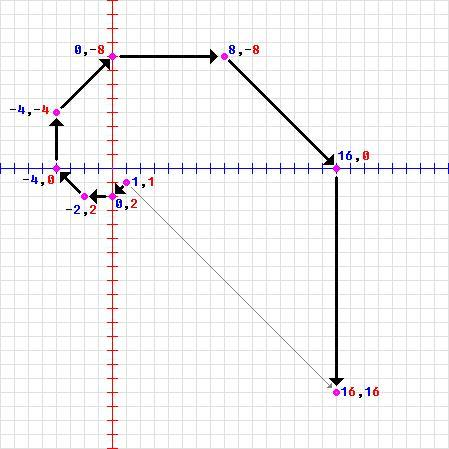Do you see that? Our equation takes us round in a circle, only, where we've ended up is further away from the centre than where we started.. That's what I meant by..

..Complex multiplication produces
a 'rotation' and a 'scaling' around the centre of the 'complex plane'..

..Let's do it again, starting with..

complex_number COMPLEX_NUMBER (1 , 1)

but then we'll..

REPEAT...8 TIMES
complex_number = (   complex_number  x COMPLEX_NUMBER (0.5 , 0.5)   )

What do we get this time?
 ..   complex_number  COMPLEX_NUMBER         (1 , 1)
 ..    complex_number  COMPLEX_NUMBER        (0 , 1)
 ..    complex_number =  COMPLEX_NUMBER   (-0.5 , 0.5 )
 ..    complex_number =  COMPLEX_NUMBER    (-0.5 , 0)
 ..    complex_number =  COMPLEX_NUMBER   (-0.25, -0.25)
 ..    complex_number =  COMPLEX_NUMBER         (0 , -0.25)
 ..    complex_number =  COMPLEX_NUMBER 0.125 , -0.125)
 ..    complex_number =  COMPLEX_NUMBER ( 0.125 , 0)
 ..    complex_number =  COMPLEX_NUMBER (0.0625 , 0.0625)

Boy!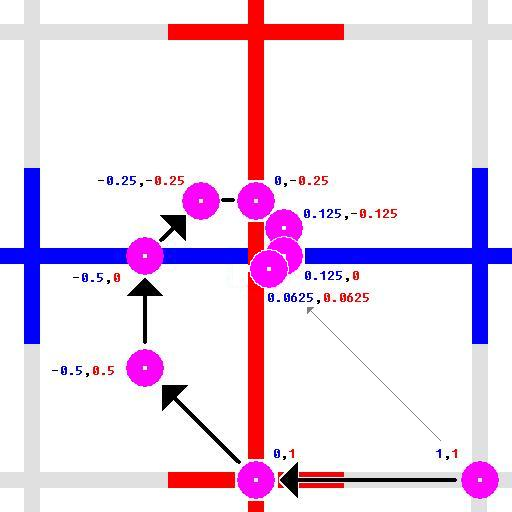D'you see that? We've gone round in a circle again, only this time we've ended up nearer to the centre than where we started from.. I bet there's a complex number that will take us round in a circle and back to where we started..

complex_number COMPLEX_NUMBER (1 , 1)
REPEAT...8 TIMES
complex_number = (   complex_number  x COMPLEX_NUMBER (0.7071 , 0.7071)   )
...
 ..   complex_number  COMPLEX_NUMBER            (1 , 1)
 ..    complex_number  COMPLEX_NUMBER           (0 , 1.4142
 ..    complex_number =  COMPLEX_NUMBER          (-1 1
 ..    complex_number =  COMPLEX_NUMBER (-1.4142 ,  0
 ..    complex_number =  COMPLEX_NUMBER          (-1 , -1 ..    complex_number =  COMPLEX_NUMBER           (0 , -1.4142 ..    complex_number =  COMPLEX_NUMBER           1 , -1
 ..    complex_number =  COMPLEX_NUMBER  ( 1.4142 , 0 ..    complex_number =  COMPLEX_NUMBER            (1 , 1
...

Well well well..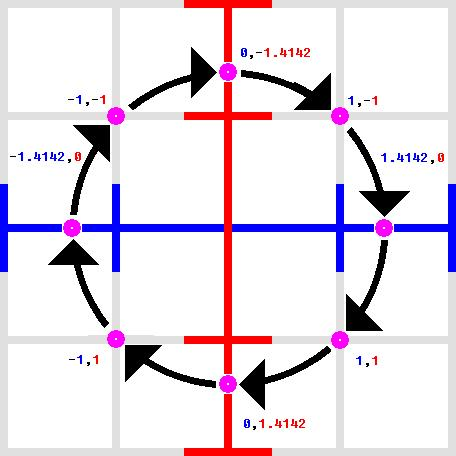For some reason, the formula for multiplying complex numbers is the same as the formula for rotating 2-D coordinates.. ..The numbers that we used (0.7071 & 0.7071) happened to correspond with trigonometrical ratios for an angle of 45 degrees, an so we went round precisely in a circle..

..sine 45 = 0.7071 .. cosine 45 = 0.7071 ..

[4..] Pictures From Numbers..

See how some complex numbers can move away from the centre when we multiply them?

Well, what we do is..

..we draw a circle around the centre of the complex plane..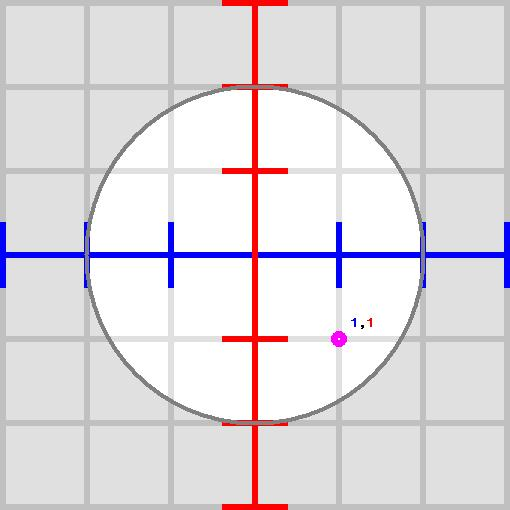..and then we count just how long it takes for our equation..

E.G., complex_number = (  complex_number x COMPLEX_NUMBER (1 , 1)  )

..to 'throw' our complex number outside of that circle...

.If it takes too long then we stop..
...
Using coordinates from all over the screen as starting points..
count                    = 0
complex_number = COORDINATE(left_and_right_number , up_and_down_number)
REPEAT..
count                     = count + 1
complex_number
=
(   complex_number  x COMPLEX_NUMBER (1 , 1)   )
..UNTIL complex_number IS TOO BIG
OR UNTIL count IS TOO BIG
..
colour = count
..you get a picture like this..(..remember, BLACK = '0'; MAROON = '1'; GREEN = '2'; etc..)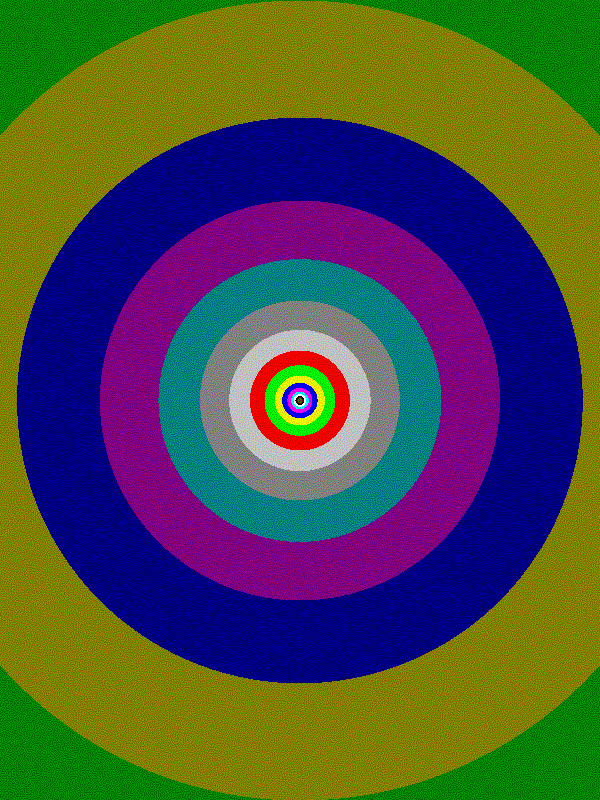[..Flora_04a..]

Changing the equation very slightly to..

complex_number
=  (  complex_number  x COMPLEX_NUMBER (1 , 1)  )  +  COMPLEX_NUMBER (0.5 , 0.5)

..produces a graph like this..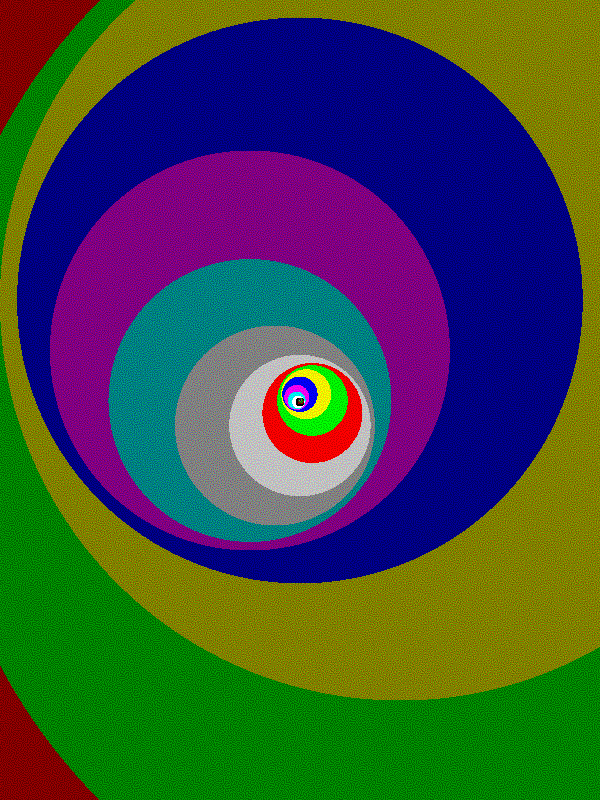[..Flora_04b..]

Change the equation to..

complex_number
=  (  complex_number x complex_number  )  +  COMPLEX_NUMBER (2 , 0)

..and you get this..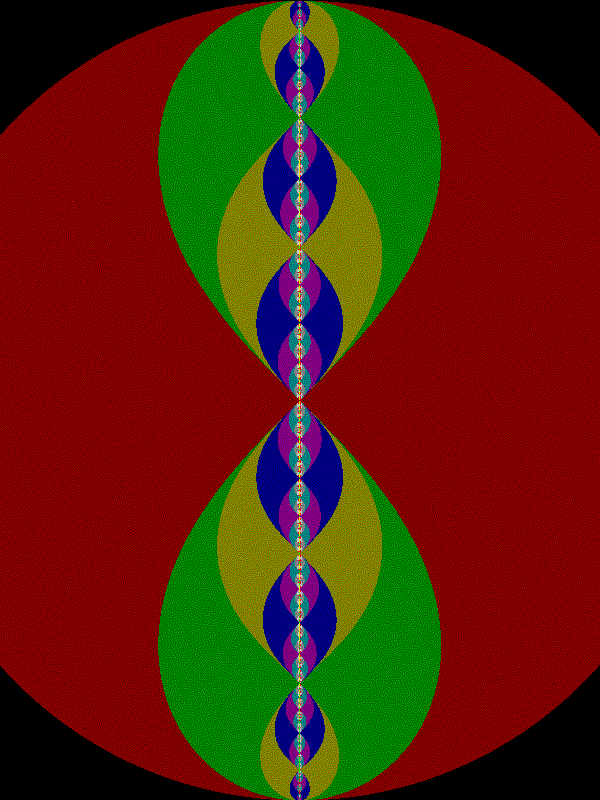Wow.. Cool huh?